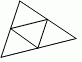Competitions

# Triangles

Time limit 1 second
Memory limit 128 MiB

Mike likes to draw triangles, but he does it in an unusual way. First he draws an arbitrary triangle, then divides each side into n equal parts and draws through the partition points the lines parallel to sides of triangle. As a result he gets a number of triangles equal to each other. Help Mike to find the maximum number of identical triangles in his final picture.## Input data

One integer n (0 < n < 2 * 10^9).

## Output data

The maximum number of triangles equal to each other.

## Examples

Input example #1
2

Output example #1
4